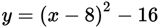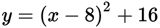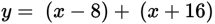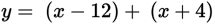# SAT Math Multiple Choice Question 926: Answer and Explanation

### Test Information

Question: 926

10. Etienne is graphing the quadratic equation y = x2 – 8x – 48. He substitutes 0 for x and finds that the y-intercept of the graph is –48. Next, he wants to plot the x-intercepts of the graph, so he rewrites the equation in a different form. Assuminghe rewrote the equation correctly and the equation reveals the x-intercepts, which of the following is Etienne's new equation?

• A.• B.• C.• D.